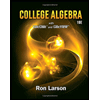Question

63% of all students at a college still need to take another math class. If 4 students are randomly selected, find the probability that

a. Exactly 2 of them need to take another math class.
b. At most 2 of them need to take another math class.
c. At least 2 of them need to take another math class.
d. Between 2 and 3 (including 2 and 3) of them need to take another math class.

Round all answers to 4 decimal places.

Expert Solution

### Want to see the full answer?

Check out a sample Q&A hereStudents who’ve seen this question also like:College Algebra
10th Edition
ISBN: 9781337282291
Author: Ron Larson
Publisher: Cengage Learning